## 预习报告

### 内容一

74LS194 芯片相关。

#### 功能与真值表

CP 时钟$\overline{Cr}$清零${S_1}$${S_0}工作状态Q_A^+$$Q_B^+$$Q_C^+$$Q_D^+$
$\uparrow$0XX置零0000
$\uparrow$100保持$Q_A$$Q_B$$Q_C$$Q_D \uparrow101右移D_{SR}$$Q_A$$Q_B$$Q_C$
$\uparrow$110左移$Q_B$$Q_C$$Q_D$$D_{SL} \uparrow111并行送数D_0$$D_1$$D_2$$D_3$

### 内容二

#### 原理

1. 利用 194 右移功能，实现「0」向右边移动；同时利用 JK 触发器翻转功能（J=1，K=1），控制 194 从右移变为并行送数，实现$1110\to0111$的变化。
2. 如果没有先清零，194 的 S1 输入不确定，可能会出现输出 1111 的情况（CP 触发不变化），也可能是其他异常。

#### 用 Proteus 设计电路图，并运行仿真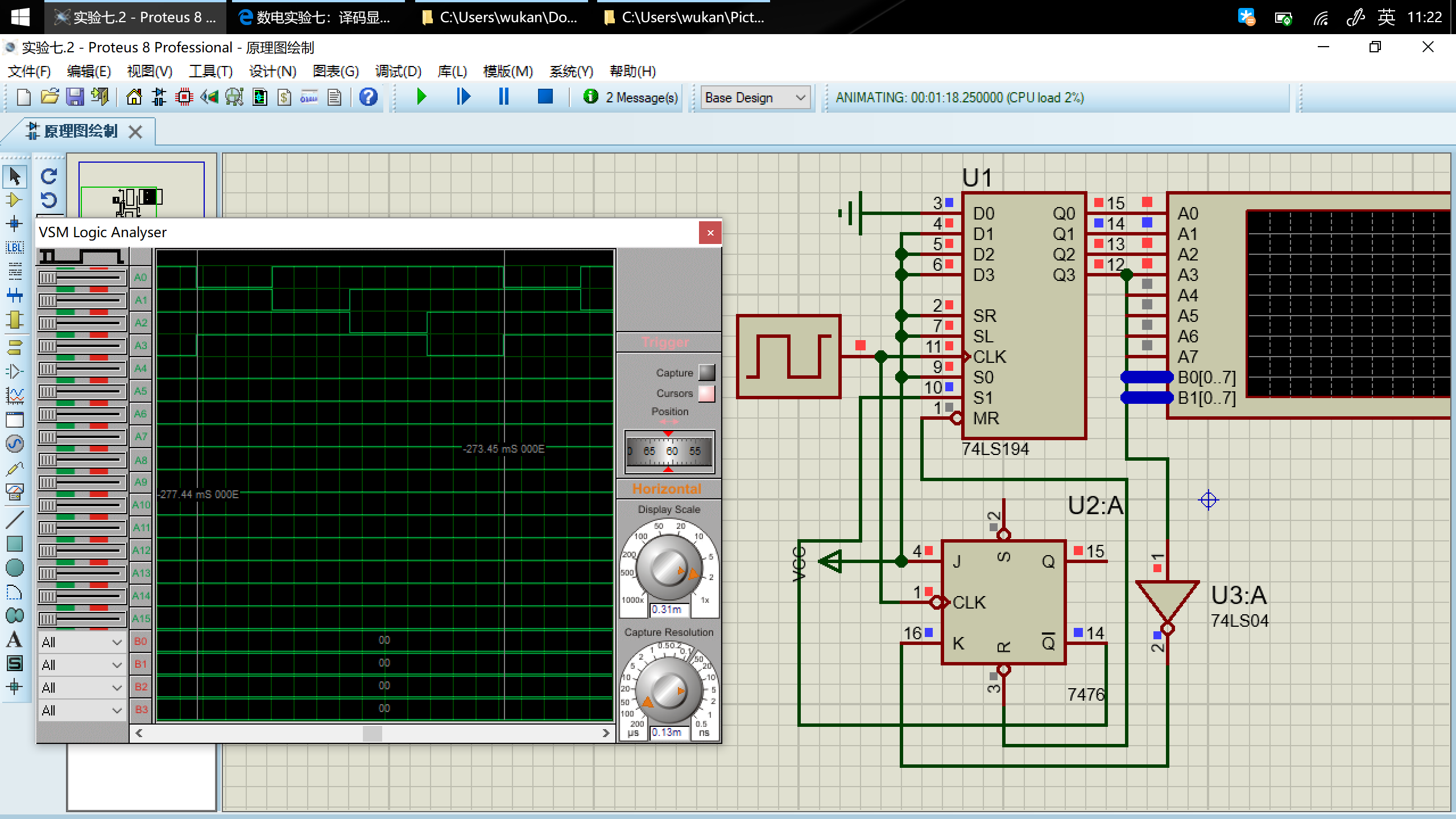如图，$Q_0Q_1Q_2Q_3$的变化为

$0111\to1011\to1101\to1110\to0111\to\ldots$

### 内容四

#### 方法一：显示位置决定显示内容

##### 用 Proteus 设计电路图，并运行仿真#### 方法二：显示内容决定显示位置

##### 用 Proteus 设计电路图，并运行仿真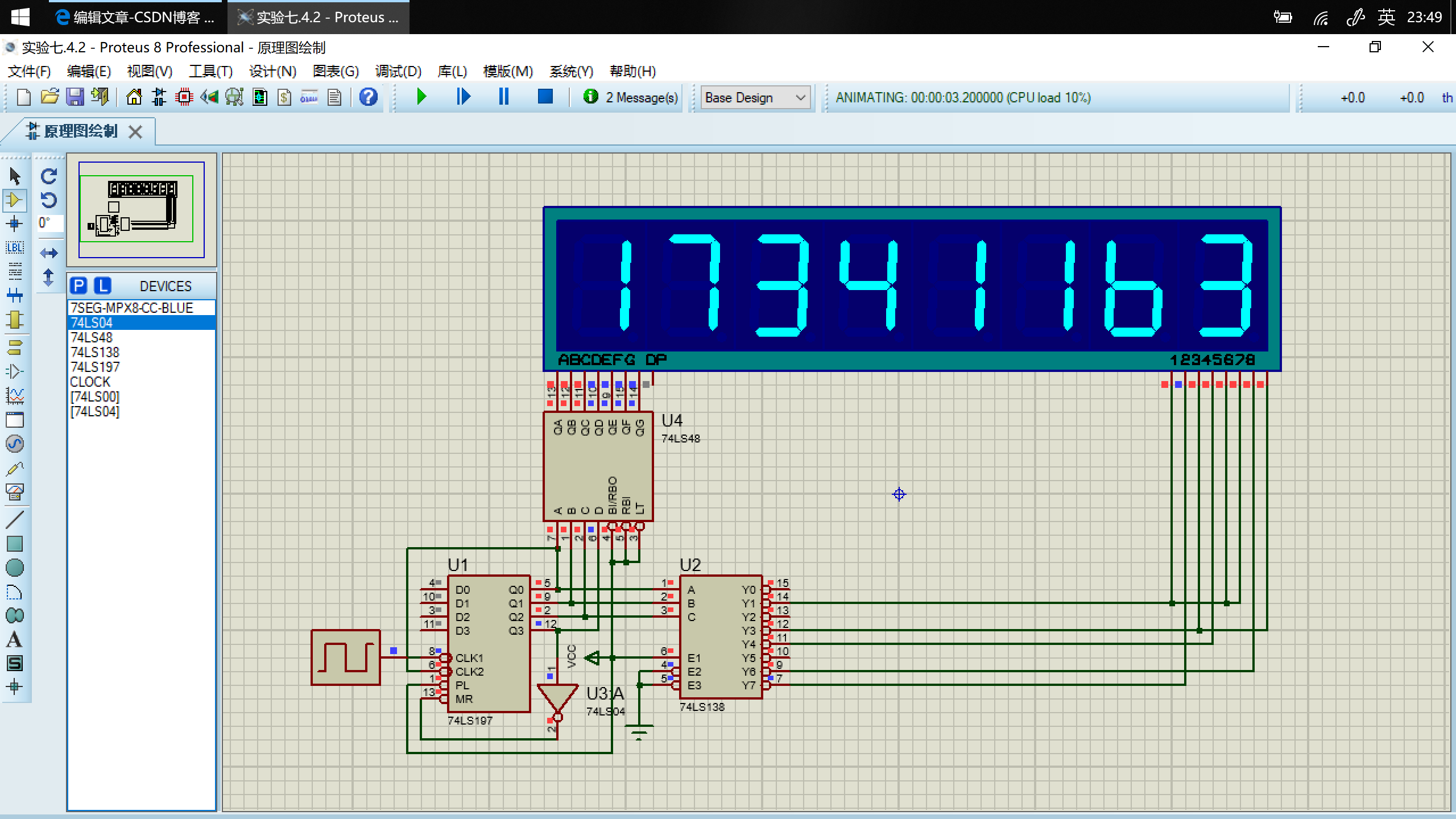### 内容五

#### 原理

8*8 点阵由 64 个发光二极管组成，当二极管所在位置的行电平为高，列电平为低时，相应的二极管就被点亮。与 7 段数码管结构比较可以看出，实验箱点阵每一行可以看成是一组共阳极数码管，每一列可以看成是一组共阴极数码管。

#### 真值表

$Q_2$$Q_1$$Q_0$$R_1$$R_2$$R_3$$R_4$$R_5$$R_6$$R_7$$R_8$
00000100010
00101111100
01000100100
01100010010
10001100100
10100111000
11000100100
11100110010

#### Proteus 设计电路图并仿真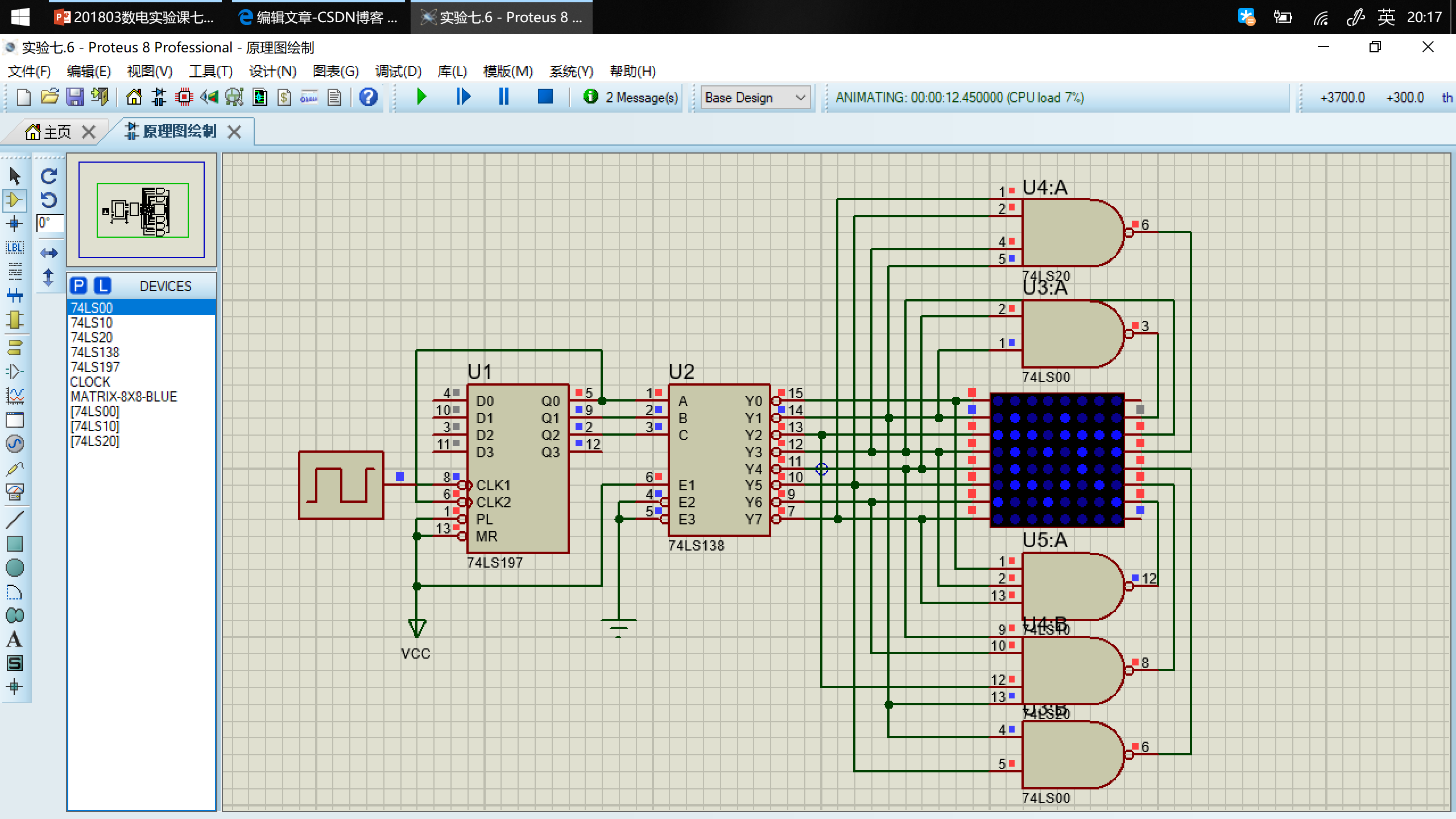### 内容六（加分项）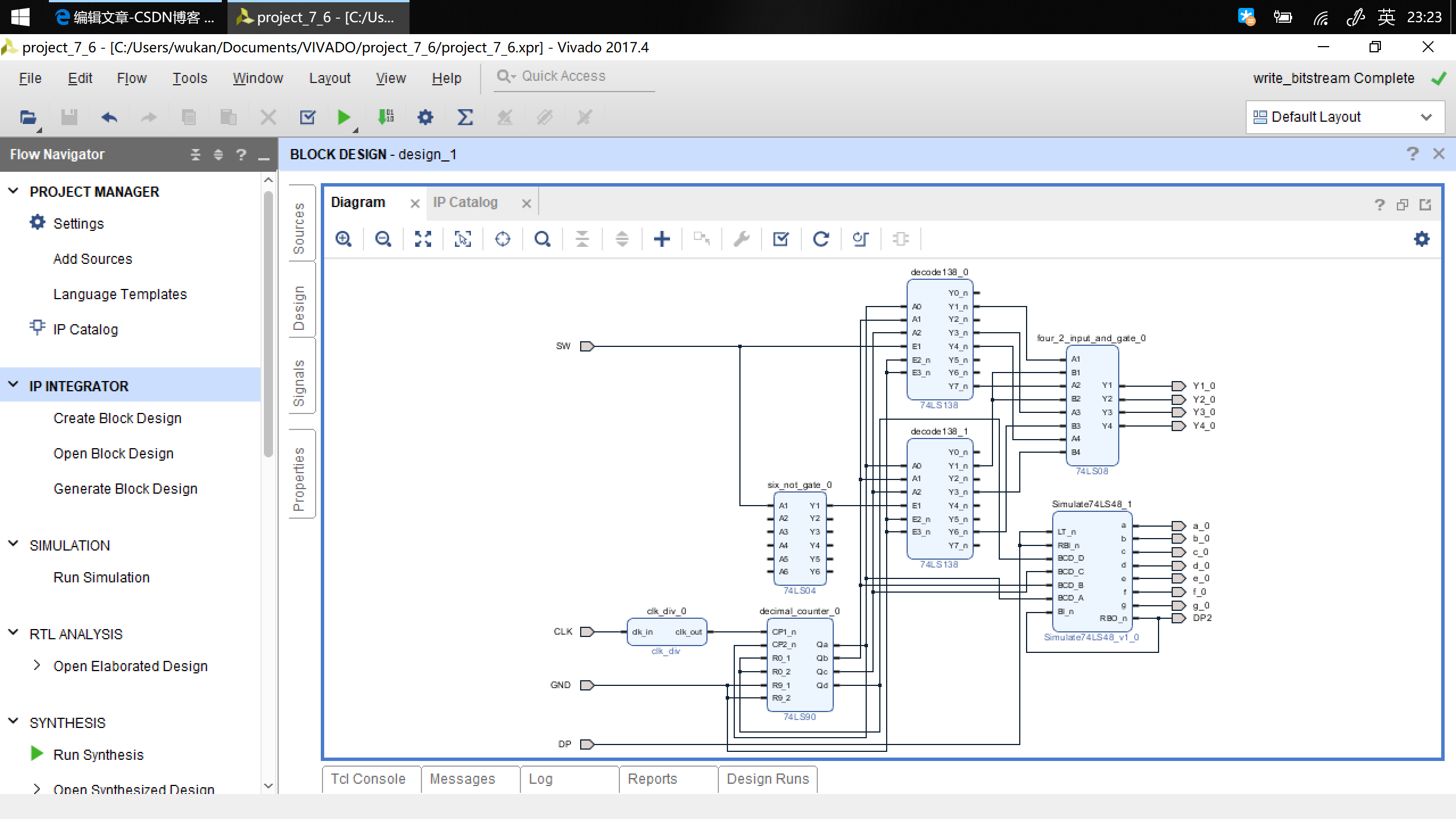#### 端口映射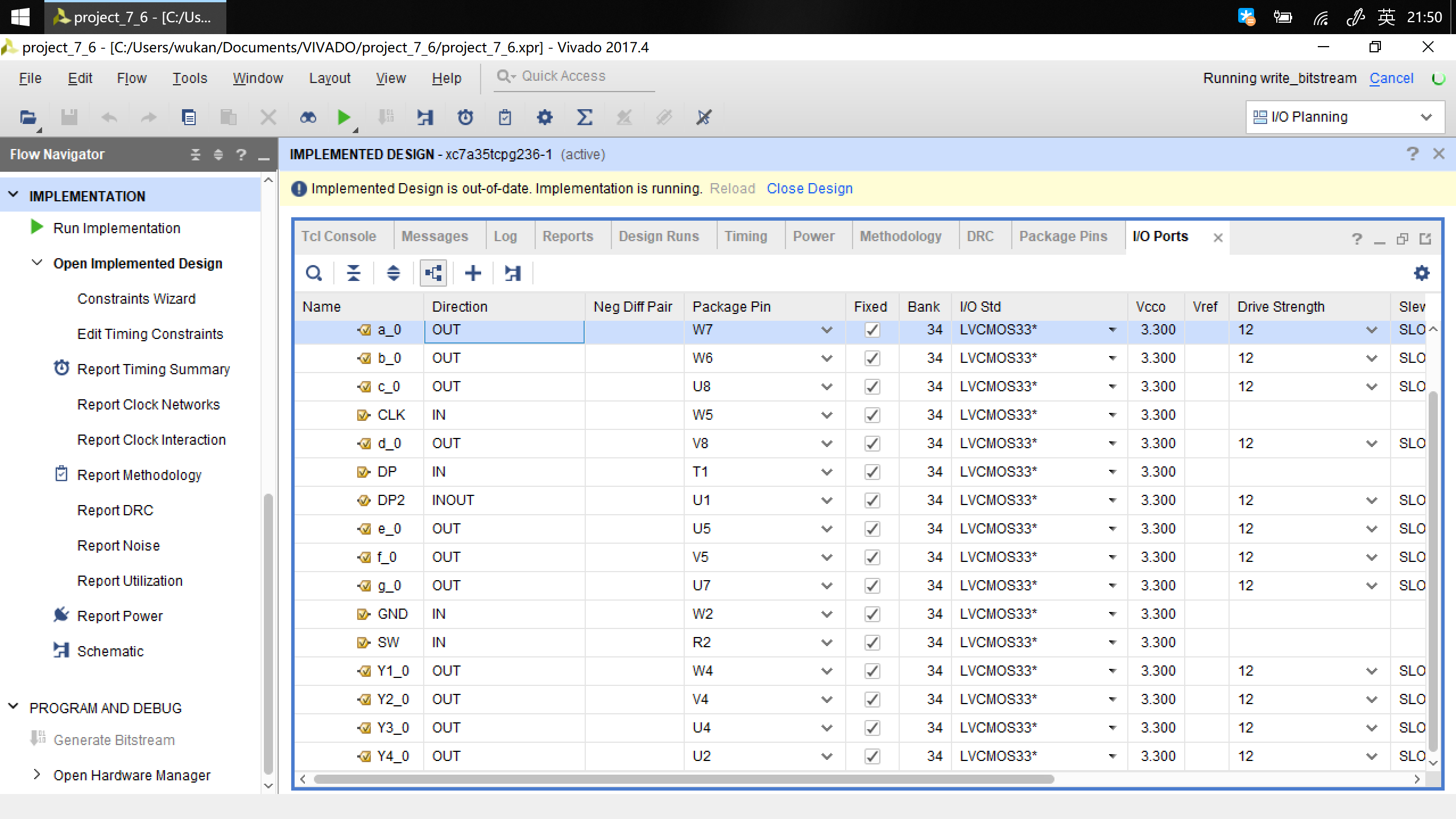#### 烧写到 Basys3 实验板上，检查效果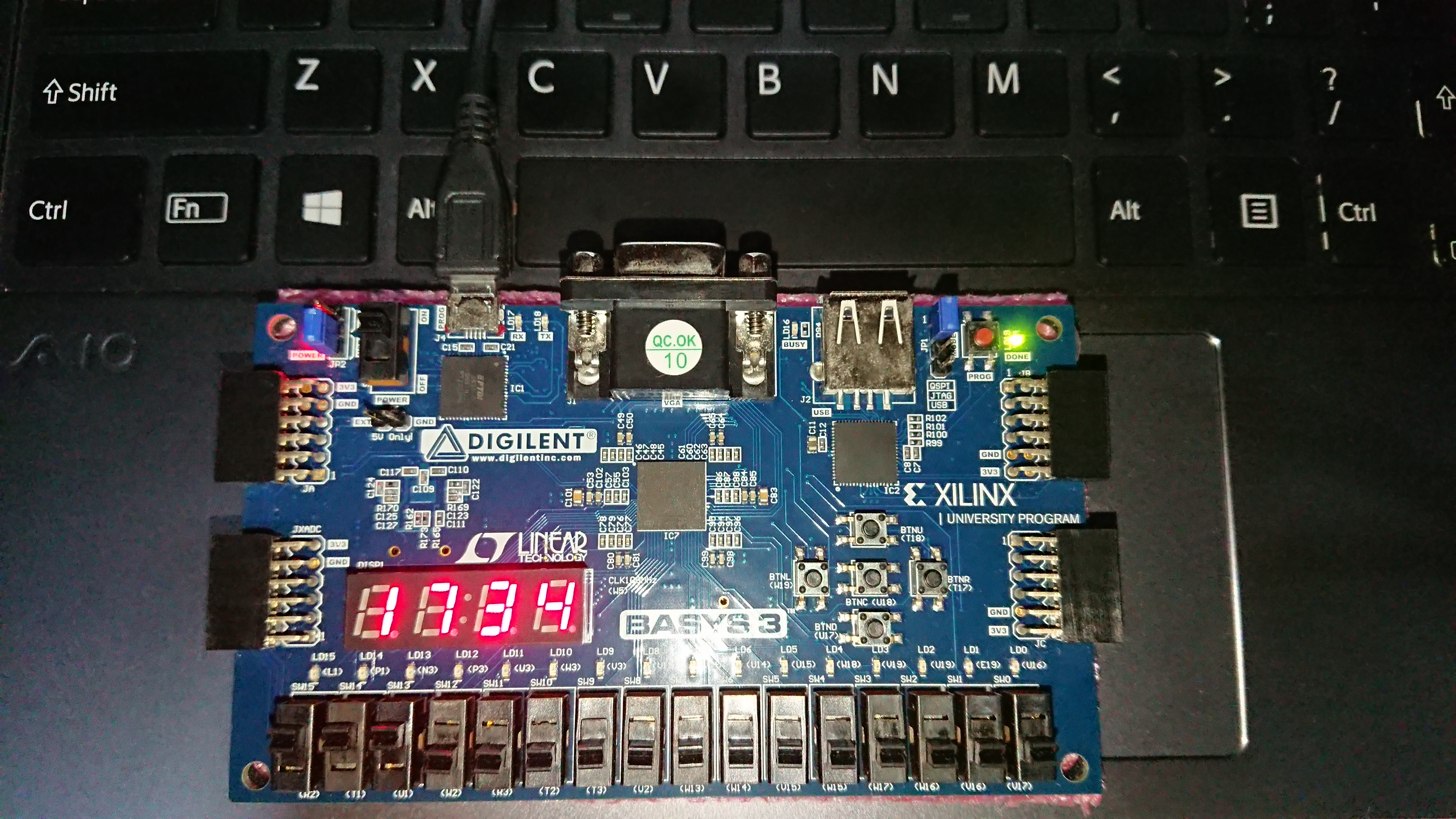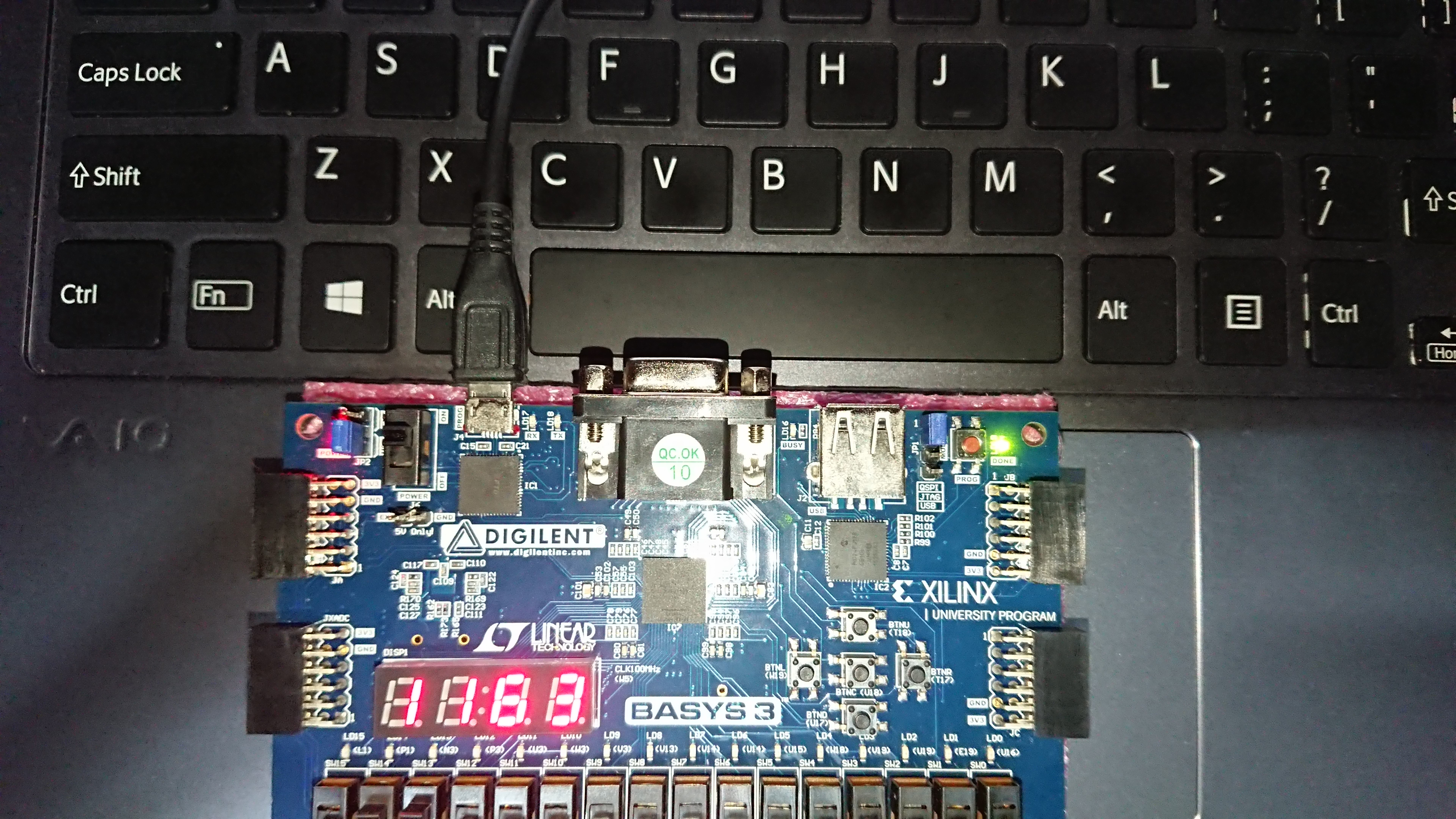## 实验报告

### 内容三

#### 原理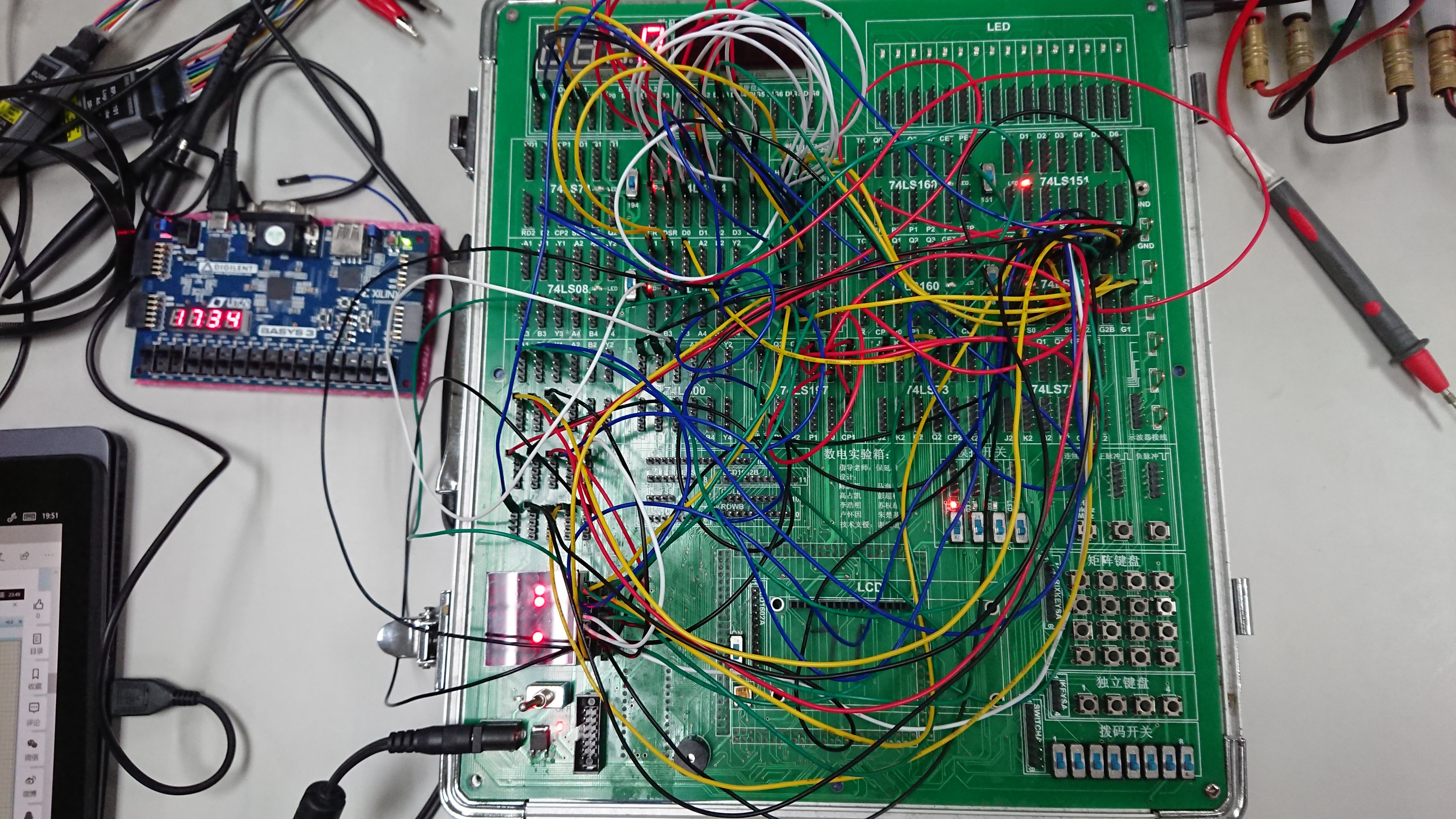### 内容五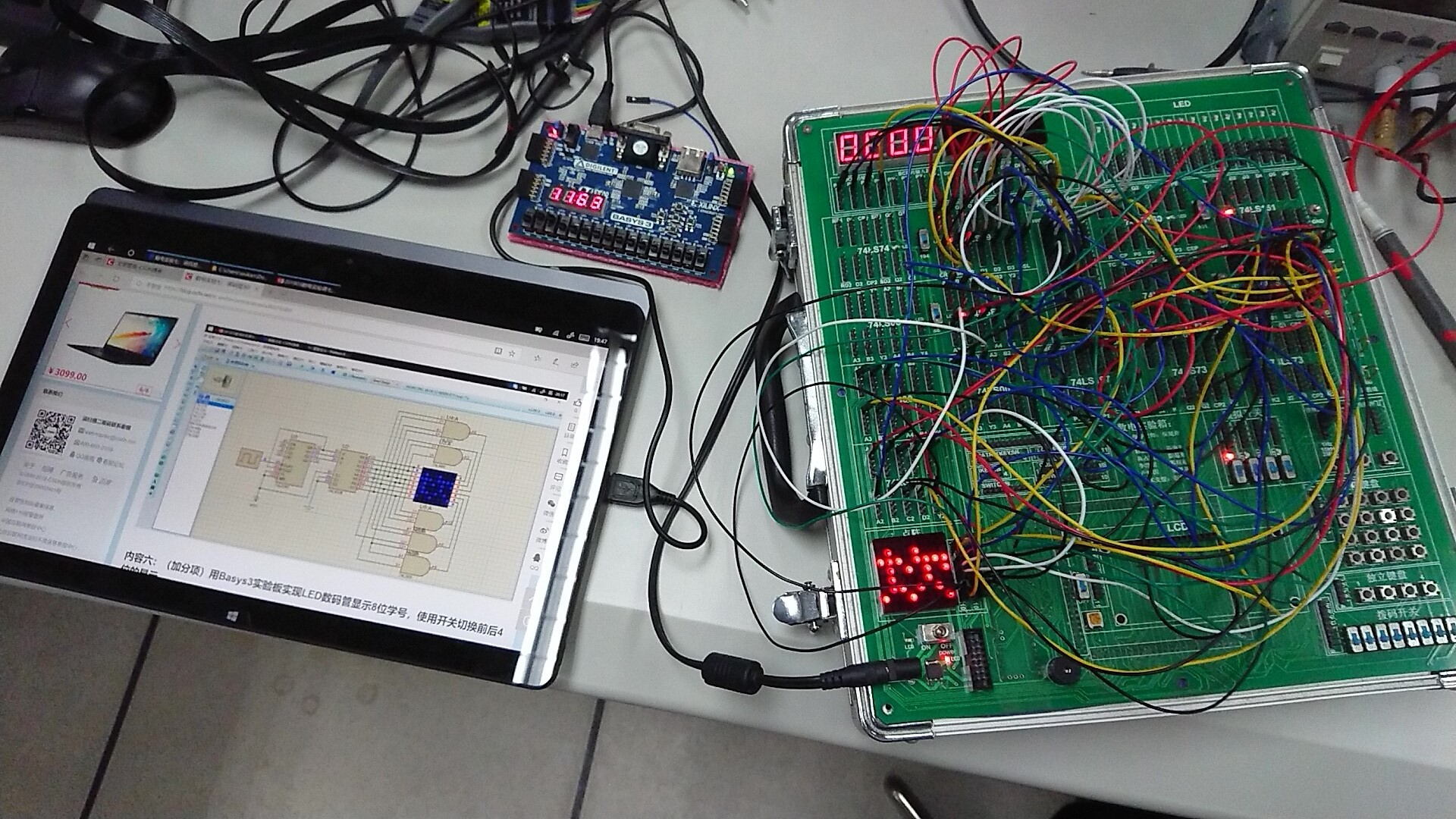### 实验心得与体会

1. 在点阵显示「坎」的实验中，接线较为复杂，而刚接完时只有第一列灯亮，经过排查发现是 74LS197 清零端接错。锻炼了自己接线的熟练度，并学会逐步排查电路故障。
2. 由于实验箱上非门和四输入与非门的数量不够，使用了 74LS86（异或）和 74LS00（双输入与非门）代替，学会在找不到原有元件下如何利用其它元件组合来达到预期效果。